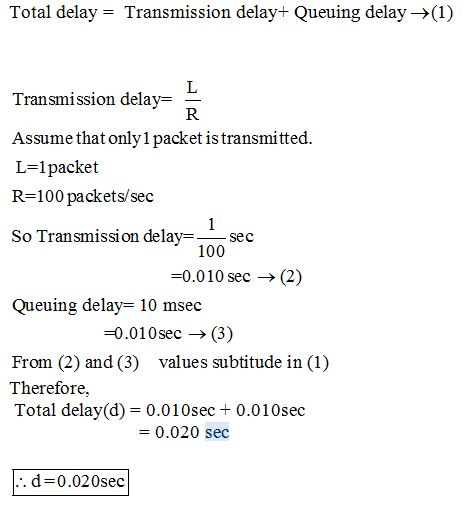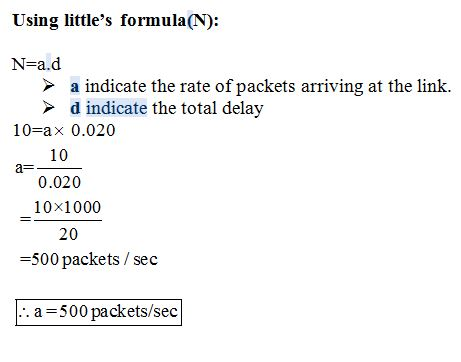SHARE
HELP

To start with, Sr2Jr’s first step is to reduce the expenses related to education. To achieve this goal Sr2Jr organized the textbook’s question and answers. Sr2Jr is community based and need your support to fill the question and answers. The question and answers posted will be available free of cost to all.Authors:
James F. Kurose, Keith W. Ross
Chapter:
Computer Networks And The Internet
Exercise:
Problems
Question:16 | ISBN:9780132856201 | Edition: 6

###Question

Consider a router buffer preceding an outbound link. In this problem, you will use Little’s formula, a famous formula from queuing theory. Let N denote the average number of packets in the buffer plus the packet being transmitted. Let a denote the rate of packets arriving at the link. Let d denote the average total delay (i.e., the queuing delay plus the transmission delay) experienced by a packet. Little’s formula is N = a · d. Suppose that on average, the buffer contains 10 packets, and the average packet queuing delay is 10 msec. The link’s transmission rate is 100 packets/sec. Using Little’s formula, what is the average packet arrival rate, assuming there is no packet loss?

###AnswerTherefore, the average packet arrival rate is 500 packets/sec

### Discussions

Post the discussion to improve the above solution.